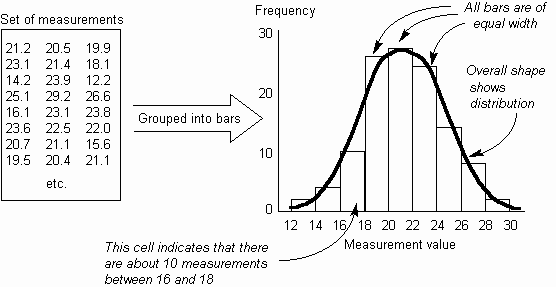The Psychology of Quality and More

# Histogram

When measuring a process, it often occurs that the measurements vary within a range of values. By understanding how these measurements vary, the effects of the process and changes made to it can be better understood.

The Histogram shows the frequency distribution across a set of measurements as a set of physical bars. The width of each bar is constant and represents a fixed range of measurements (called a cell, bin or class). The height of each bar is proportional to the number of measurements within that cell. Each bar gives a solid visual impression of the number of measurements in it and together the bars show the distribution across the measurement range. The distribution of measurements can be seen far more clearly in the Histogram than in a table of numbers.Variation, Sturgess Rule

Toolbook chapter: Histogram

### You can buy books here

And the big
paperback book